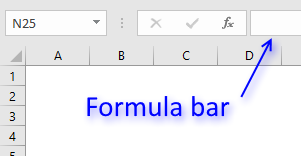Author: Oscar Cronquist Article last updated on February 01, 2019

Problem: Find the smallest value in a list but it has to be bigger than 45?

Answer:

1. MINIFS function [Excel 2016]
2. MIN + IF functions [Excel 2013 and previous versions]

### MINIFS function [Excel 2016]

Formula in cell D6:

=MINIFS(B3:B16,B3:B16,">"&D3)

### MIN + IF functions [Array Formula]

Array formula in cell D6:

=MIN(IF(B3:B16>D3,B3:B16))

To enter an array formula, type the formula in cell B3 then press and hold CTRL + SHIFT simultaneously, now press Enter once. Release all keys.The formula bar now shows the formula with a beginning and ending curly bracket telling you that you entered the formula successfully.

Don't enter the curly brackets yourself, they appear automatically.

### Explaining array formula

#### Step 1 - Filter values in cell range larger than the condition in cell D3

The IF function allows you to create a logical expression that evaluates to TRUE or FALSE. You can then choose what will happen to a value that returns TRUE and also FALSE.

IF(logical_test, [value_if_true], [value_if_false])

If you use a cell range instead of a cell reference to a single cell you evaluate all the values in the cell range. The larger than sign lets you check if the values in B3:B16 are larger than the value in D3.

IF(B3:B16>D3,B3:B16)

becomes

IF({86;51;68;50;38;66;23;59;79;72;19;99;76;88}>45,B3:B16)

becomes

IF({TRUE; TRUE; TRUE; TRUE; FALSE; TRUE; FALSE; TRUE; TRUE; TRUE; FALSE; TRUE; TRUE; TRUE},B3:B16)

becomes

IF({TRUE; TRUE; TRUE; TRUE; FALSE; TRUE; FALSE; TRUE; TRUE; TRUE; FALSE; TRUE; TRUE; TRUE},{86; 51; 68; 50; 38; 66; 23; 59; 79; 72; 19; 99; 76; 88})

and returns the following array:

{86;51;68;50;FALSE;66;FALSE;59;79;72;FALSE;99;76;88}

#### Step 2 - Return the smallest value in the array

The MIN function ignores boolean values which is useful in this case.

MIN(IF(B3:B16>D3,B3:B16))

becomes

MIN({86;51;68;50;FALSE;66;FALSE;59;79;72;FALSE;99;76;88})

and returns 50 in cell D6.